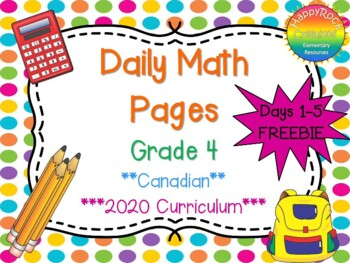# Grade 4 Daily Math Days 1-5 Freebie

Rated 4.85 out of 5, based on 20 reviews
20 Ratings;
4th
Subjects
Resource Type
Formats Included
• PDF
Pages
10 pages
Report this resource to TPT

### Description

Do you want an easy way to cover all of the math curriculum expectations, help your students see connections between math strands AND improve their skills and computation? This is the resource you have been looking for!!!

These daily math pages focus on a variety of skills, topics and math facts - they are easy to use, versatile and can be the perfect math warm up, bell work or homework pages. freebythesea

What is Included?

These daily math pages include a variety of questions from all math strands - perfect for spiraling or review!  Each day includes 9 short answer questions OR 1-2 rich problem solving tasks. I use these at the start of every math class and have seen a huge improvement in my students being able to make connections between strands and retain concepts throughout the year.  Teachers have commented that they use these pages for bell work, homework and are perfect for differentiating!

Each package contains 20 days of math (9 short answer questions daily OR a rich problem solving task). There is an answer page for each day. I simply project the questions on my screen, my kiddos solve them on a whiteboard and then share their answers. My kids love being the teacher - asking their peers for the answers and modelling how to answer each question. Alternatively, you can print a copy for each student, or print a few copies, place them in plastic sheets and use them as a math center.

What Topics Are Covered? (NEW 2020 Curriculum)

NUMBER

• addition and subtraction questions on every page (4 digits), plus tenths
• representing numbers to 10 000
• place value to 10 000
• composing and decomposing 4 digit numbers
• reading, representing and comparing decimal tenths
• rounding decimal numbers to the nearest whole number
• counting forwards by halves, thirds, fourths, sixths, eighths and tenths
• represent fractions from halves to tenths
• equivalent fractions and decimals
• multiplying fractions with same denominators
• rounding to the nearest 10, 100 and 1000
• multiplication to 10 x 10 and division to 100/10
• multiplying and dividing 2 and 3 digit numbers by 1 digit

ALGEBRA

• repeating patterns
• growing patterns
• equivalent expressions
• introduction to variables
• equations to 50
• inequalities to 20

DATA

• qualitative data vs quantitative data
• primary and secondary sources
• comparing data using frequency tables and stem and leaf plots
• display sets of data using a variety of graphs
• create an infographic about a data set
• interpreting and analyzing data (including identifying the mode, mean and median)
• graphs
• mathematical language related to probability (impossible, unlikely, equally likely, likely, certain) using probability lines

SPATIAL SENSE

• geometric properties of rectangles (right angles, parallel and perpendicular sides, lines of symmetry)
• plot and read co-ordinates on the first quadrant of a Cartesian plane
• describe and perform translations and reflections on a grid
• explain relationship between grams/kilograms and liters/milliliters
• use metric prefixes to describe the relative size of metric units and choose appropriate units to measure length, mass and capacity
• elapsed time
• identify angles (right, acute, obtuse, straight)
• use row/column of an array to measure areas of rectangles
• apply formula for the area of a rectangle to find unknown measurements

FINANCIAL LITERACY

• identify various methods of payment
• estimate the cost of transactions and change (no sales tax)
• explain concepts of spending, saving, earning, investing and donating
• describe ways of determining whether something is reasonably priced

This resource does not cover expectations from strand A (Social-Emotional Learning Skills) or C3 (Coding)

Updated August 2020 to new 2020 math curriculum!

"This helped me gain a lot of valuable time with my all my students and a chance to reach some of my students who have math issues in a fun way. Thanks!"

"I used this resource for homework and it was very popular with both students are parents. Provides great review of important concepts and is clear and organized. I will be purchasing the grade 3 this year. Thank you!"

"I use this every morning with my grade 4s and love it!!"

"Excellent! Thank you for such a well made, highly engaging and useful resource!!!"

These daily math pages include a variety of questions from all math strands - perfect for spiraling or review!  Each day includes 9 short answer questions OR 1-2 rich problem solving tasks. I use these at the start of every math class and have seen a huge improvement in my students' ability to make connections between strands and retain concepts throughout the year.  Teachers have commented that they use these pages for bell work, homework and are perfect for differentiating!

Check out the preview or Daily Math Grade 4 Days 1-5 Freebie

Be sure to follow my store HappyRock Creations for more great resources!

*Please note that this product uses Canadian units and currency, and is based on the Ontario math curriculum.*

Total Pages
10 pages